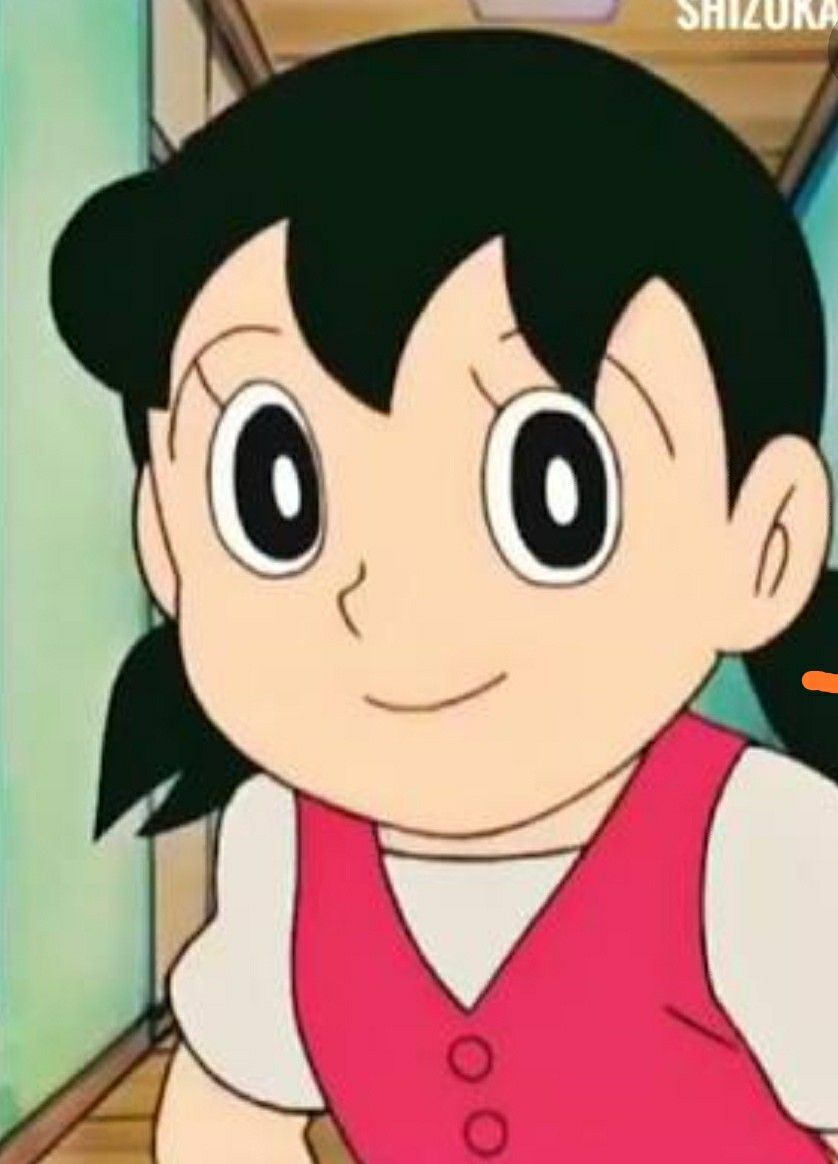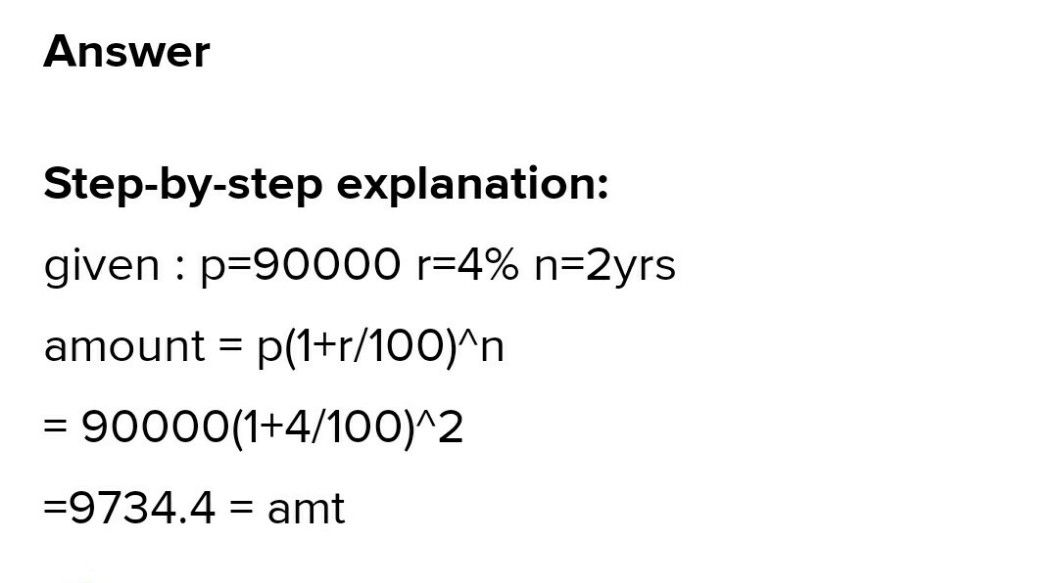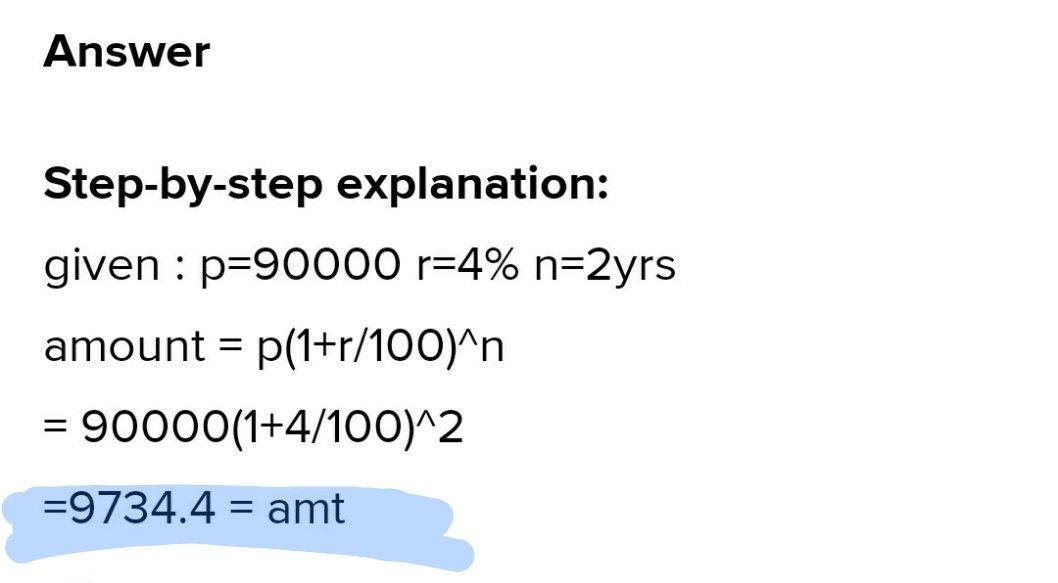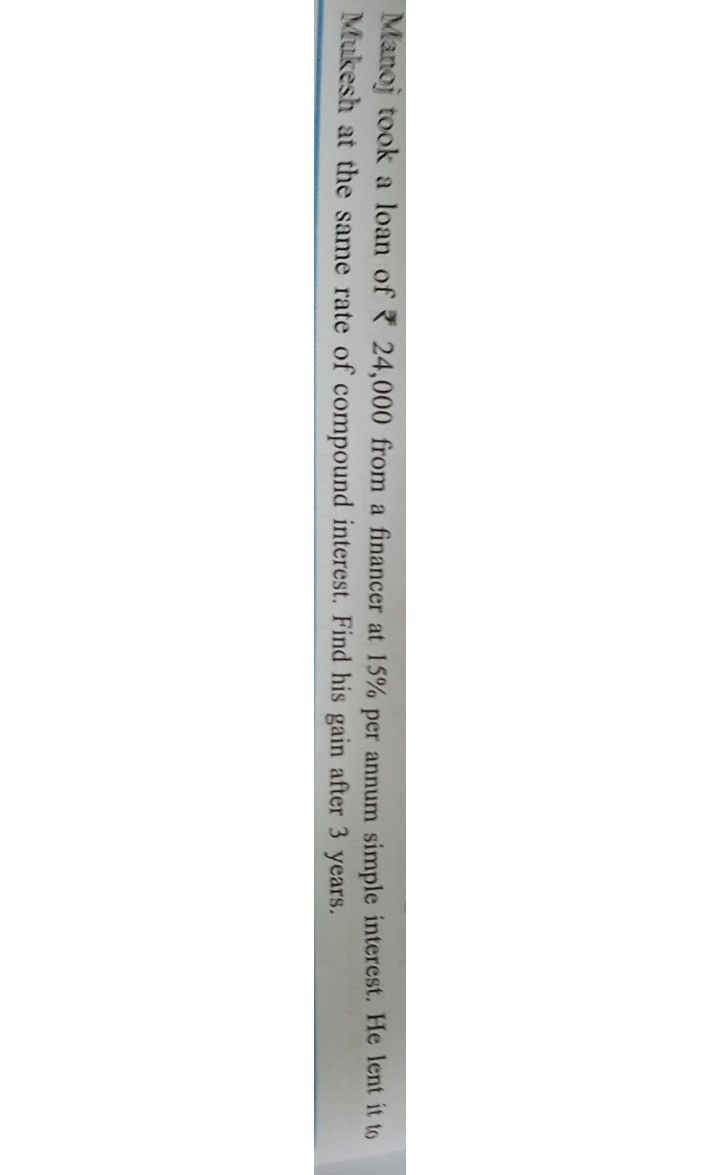Symbol
Problem$4.$ Calculate the amount and compound interest on $x$ $90,000$ at the rate of $40%$ $P.$ p.a. $1.$ compounded annually for $2$ years.
7th-9th grade
Algebra
Search count: 110
SolutionQanda teacher - askSHIZUKAask if doubt dear
u can ask more such questions
in preffered matchStudent
Amount =97344 , Interest =7344
yeh hai answer
photo send kijeye
kya hua missQanda teacher - askSHIZUKAye lijiye beta
answerStudent
kya lijeye
kisko beta bol rhe hoQanda teacher - askSHIZUKA
dear student
i have shared you answerStudent
hlo
i have one question
please solve
hlo
hlo
hlo
i have one question
please solveQanda teacher - askSHIZUKA
yes
askStudentkya hua
nahi bana
bana gya toh pic send kijeye
hlo
hlo
hlo
hlo
hlo
kya hua
miss
hlo
ban gaya kya
missQanda teacher - askSHIZUKA
please dear student
ask one question at once